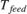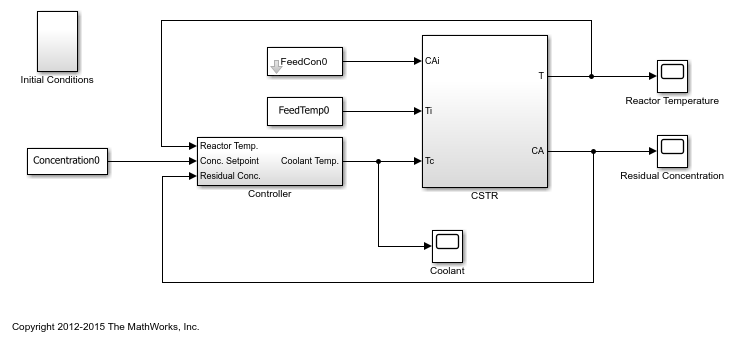# Design Exploration Using Parameter Sampling (Code)

This example shows how to sample and explore a design space. You explore the design of a Continuously Stirred Tank Reactor to minimize product concentration variation and production cost. The design includes feed stock uncertainty.

You explore the CSTR design by characterizing design parameters using probability distributions. You use the distributions to generate random samples in the design space and perform Monte-Carlo evaluation of the design at these sample points. You then create plots to visualize the design space and select the best design. You can then use the best design as an initial guess for optimization of the design.

You can also use the sampled design space and Monte-Carlo evaluation output to analyze the influence of design parameters on the design; see Identify Key Parameters for Estimation (Code).

### Continuously Stirred Tank Reactor (CSTR) Model

Continuously Stirred Tank Reactors (CSTRs) are common in the process industry. The Simulink® model, sdoCSTR, models a jacketed diabatic (i.e., non-adiabatic) tank reactor described in . The CSTR is assumed to be perfectly mixed, with a single first-order exothermic and irreversible reaction,., the reactant, is converted to, the product.

In this example, you use the following two-state CSTR model, which uses basic accounting and energy conservation principles:•, and- Concentrations of A in the CSTR and in the feed [kgmol/m^3]

•,, and- CSTR, feed, and coolant temperatures [K]

•and- Volumetric flow rate [m^3/h] and the density of the material in the CSTR [1/m^3]

•and- Height [m] and heated cross-sectional area [m^2] of the CSTR.

•- Pre-exponential non-thermal factor for reaction[1/h]

•and- Activation energy and heat of reaction for[kcal/kgmol]

•- Boltzmann's gas constant [kcal/(kgmol * K)]

•and- Heat capacity [kcal/K] and heat transfer coefficients [kcal/(m^2 * K * h)]

open_system('sdoCSTR');### CSTR Design Problem

Assume that the CSTR is cylindrical, with the coolant applied to the base of the cylinder. Tune the CSTR cross-sectional area,, and CSTR height,, to meet the following design goals:

• Minimize the variation in residual concentration,. Variations in the residual concentration negatively affect the quality of the CSTR product. Minimizing the variations also improves CSTR profit.

• Minimize the mean coolant temperature. Heating or cooling the jacket coolant temperature is expensive. Minimizing the mean coolant temperature improves CSTR profit.

The design must allow for variations in the quality of supply feed concentration,, and feed temperature,. The CSTR is fed with feed from different suppliers. The quality of the feed differs from supplier to supplier and also varies within each supply batch.

### Specify Design Variables

Select the following model parameters as design variables:

• Cylinder cross-sectional area• Cylinder heightp = sdo.getParameterFromModel('sdoCSTR',{'A','h'}); 

Limit the cross-sectional area to a range of [0.2 2] m^2.

p(1).Minimum = 0.2; p(1).Maximum = 2; 

Limit the height to a range of [0.5 3] m.

p(2).Minimum = 0.5; p(2).Maximum = 3; 

### Sample the Design Space

Create a parameter space for the design variables. The parameter space characterizes the allowable parameter values and combinations of parameter values.

pSpace = sdo.ParameterSpace(p); 

The parameter space uses default uniform distributions for the design variables. The distribution lower and upper bounds are set to the design variable minimum and maximum value respectively.

Use the sdo.sample function to generate samples from the parameter space. You use the samples to evaluate the model and explore the design space.

rng default; % For reproducibility pSmpl = sdo.sample(pSpace,30); 

Use the sdo.scatterPlot command to visualize the sampled parameter space. The scatter plot shows the parameter distributions on the diagonal subplots and pairwise parameter combinations on the off diagonal subplots.

figure, sdo.scatterPlot(pSmpl)### Specify Uncertain Variables

Select the feed concentration and feed temperature as uncertain variables. You evaluate the design using different values of feed temperature and concentration.

pUnc = sdo.getParameterFromModel('sdoCSTR',{'FeedCon0','FeedTemp0'}); 

Create a parameter space for the uncertain variables. Use normal distributions for both variables. Specify the mean as the current parameter value. Specify a variance of 5% of the mean for the feed concentration and 1% of the mean for the temperature.

uSpace = sdo.ParameterSpace(pUnc); uSpace = setDistribution(uSpace,'FeedCon0',makedist('normal',pUnc(1).Value,0.05*pUnc(1).Value)); uSpace = setDistribution(uSpace,'FeedTemp0',makedist('normal',pUnc(2).Value,0.01*pUnc(2).Value)); 

The feed concentration is inversely correlated with the feed temperature. Add this information to the parameter space.

uSpace.RankCorrelation = [1 -0.6; -0.6 1]; 

The rank correlation matrix has a row and column for each parameter with the (i,j) entry specifying the correlation between the i and j parameters.

Sample the parameter space. The scatter plot shows the correlation between concentration and temperature.

uSmpl = sdo.sample(uSpace,60); sdo.scatterPlot(uSmpl)Ideally you want to evaluate the design for every combination of points in the design and uncertain spaces, which implies 30*60 = 1800 simulations. Each simulation takes around 0.5 sec. You can use parallel computing to speed up the evaluation. For this example you instead only use the samples that have maximum & minimum concentration and temperature values, reducing the evaluation time to around 1 min.

[~,iminC] = min(uSmpl.FeedCon0); [~,imaxC] = max(uSmpl.FeedCon0); [~,iminT] = min(uSmpl.FeedTemp0); [~,imaxT] = max(uSmpl.FeedTemp0); uSmpl = uSmpl(unique([iminC,imaxC,iminT,imaxT]) ,:) 
uSmpl = 4x2 table FeedCon0 FeedTemp0 ________ _________ 9.4555 303.58 11.175 288.13 11.293 290.73 8.9308 294.16 

### Create Evaluation Function

Create a function that evaluates the design for a given sample point in the design space. The design is evaluated on how well it minimizes the variation in residual concentration and mean coolant temperature.

Specify Design Requirements

Evaluating a point in the design space requires logging model signals. Logged signals are used to evaluate the design requirements.

Log the following signals:

• CSTR concentration, available at the second output port of the sdoCSTR/CSTR block

Conc = Simulink.SimulationData.SignalLoggingInfo; Conc.BlockPath = 'sdoCSTR/CSTR'; Conc.OutputPortIndex = 2; Conc.LoggingInfo.NameMode = 1; Conc.LoggingInfo.LoggingName = 'Concentration'; 
• Coolant temperature, available at the first output of the sdoCSTR/Controller block

Coolant = Simulink.SimulationData.SignalLoggingInfo; Coolant.BlockPath = 'sdoCSTR/Controller'; Coolant.OutputPortIndex = 1; Coolant.LoggingInfo.NameMode = 1; Coolant.LoggingInfo.LoggingName = 'Coolant'; 

Create and configure a simulation test object to log the required signals.

simulator = sdo.SimulationTest('sdoCSTR'); simulator.LoggingInfo.Signals = [Conc,Coolant]; 

Evaluation Function

Use an anonymous function with one argument that calls the sdoCSTR_design function.

evalDesign = @(p) sdoCSTR_design(p,simulator,pUnc,uSmpl); 

The evalDesign function:

• Has one input argument that specifies the CSTR dimensions

• Returns the optimization objective value

The sdoCSTR_design function uses a for loop that iterates through the sample values specified for the feed concentration and temperature. Within the loop, the function:

• Simulates the model using the current design point, feed concentration, and feed temperature values

• Calculates the residual concentration variation and coolant temperature costs

To view the objective function, type edit sdoCSTR_design.

type sdoCSTR_design 
function design = sdoCSTR_design(p,simulator,pUnc,smplUnc) %SDOCSTR_DESIGN % % The sdoCSTR_design function is used to evaluate a CSTR design. % % The |p| input argument is the vector of CSTR dimensions. % % The |simulator| input argument is a sdo.SimulinkTest object used to % simulate the |sdoCSTR| model and log simulation signals. % % The |pUnc| input argument is a vector of parameters to specify the CSTR % input feed concentration and feed temperature. The |smplUnc| argument is % a table of different feed concentration and temperature values. % % The |design| return argument contains information about the design % evaluation that can be used by the |sdo.optimize| function to optimize % the design. % % see also sdo.optimize, sdoExampleCostFunction % % Copyright 2012-2013 The MathWorks, Inc. %% Model Simulations and Evaluations % % For each value in |smplUnc|, configure and simulate the model. Use % the logged concentration and coolant signals to compute the design cost. % costConc = 0; costCoolant = 0; for ct=1:size(smplUnc,1) %Set the feed concentration and temperature values pUnc(1).Value = smplUnc{ct,1}; pUnc(2).Value = smplUnc{ct,2}; %Simulate model simulator.Parameters = [p; pUnc]; simulator = sim(simulator); logName = get_param('sdoCSTR','SignalLoggingName'); simLog = get(simulator.LoggedData,logName); %Compute Concentration cost based on the standard deviation of the %concentration from a nominal value. Sig = find(simLog,'Concentration'); costConc = costConc+10*std(Sig.Values-2); %Compute coolant cost based on the mean deviation from room %temperature. Sig = find(simLog,'Coolant'); costCoolant = costCoolant+abs(mean(Sig.Values - 294))/30; end %% Return Total Cost % % Compute the total cost as a sum of the concentration and coolant costs. % design.F = costConc + costCoolant; %% % Add the individual cost terms to the return argument. These are not used % by the optimizer, but included for convenience. design.costConc = costConc; design.costCoolant = costCoolant; end 

### Evaluate

Use the sdo.evaluate command to evaluate the model at the sample design points.

y = sdo.evaluate(evalDesign,p,pSmpl); 
Model evaluated at 30 samples. 

View the results of the evaluation using a scatter plot. The scatter plot shows pairwise plots for each design variable (A,h) and design cost. The plot includes the total cost, F, as well as coolant and concentration costs, costCoolant and costConc respectively.

sdo.scatterPlot(pSmpl,y);The plot shows that larger cross-sectional areas result in lower total costs. However it is difficult to tell how the height influences the total cost.

Create a mesh plot showing the total cost as a function of A and h.

Ftotal = scatteredInterpolant(pSmpl.A,pSmpl.h,y.F); xR = linspace(min(pSmpl.A),max(pSmpl.A),60); yR = linspace(min(pSmpl.h),max(pSmpl.h),60); [xx,yy] = meshgrid(xR,yR); zz = Ftotal(xx,yy); mesh(xx,yy,zz) view(56,30) title('Total cost as function of A and h') zlabel('Ftotal') xlabel(p(1).Name), ylabel(p(2).Name);The plot shows that high values of A and h result in lower costs. The best design in the sampled space corresponds to the design with the lowest cost value.

[~,idx] = min(y.F); pBest = [y(idx,:), pSmpl(idx,:)] 
pBest = 1x5 table F costConc costCoolant A h _____ ________ ___________ ______ ______ 2.106 1.5505 0.55552 1.9271 2.3867 

### Refine the Design Space

The total cost surface plot shows that low cost designs are designs with A in the range [1.5 2] and h in the range [2 3]. Modify the parameter space distributions for A and h and resample the design space to focus on this region.

pSpace = setDistribution(pSpace,'A',makedist('uniform',1.5,2)); pSpace = setDistribution(pSpace,'h',makedist('uniform',2,3)); pSmpl = sdo.sample(pSpace,30); 

Add the pBest found earlier to the new samples so that it is part of the refined design space.

pSmpl = [pSmpl;pBest(:,4:5)]; sdo.scatterPlot(pSmpl)Evaluate Using Refined Design Space

y = sdo.evaluate(evalDesign,p,pSmpl); 
Model evaluated at 31 samples. 

Create a mesh plot for this section of the design space. The surface indicates that better designs are near the A = 1.9, h = 2.1 point.

Ftotal = scatteredInterpolant(pSmpl.A,pSmpl.h,y.F); xR = linspace(min(pSmpl.A),max(pSmpl.A),60); yR = linspace(min(pSmpl.h),max(pSmpl.h),60); [xx,yy] = meshgrid(xR,yR); zz = Ftotal(xx,yy); mesh(xx,yy,zz) view(56,30) title('Total cost as function of A and h') zlabel('Ftotal') xlabel(p(1).Name), ylabel(p(2).Name);Find the best design from the new design space and compare with the best design point found earlier.

[~,idx] = min(y.F); pBest = [pBest; [y(idx,:), pSmpl(idx,:)]] 
pBest = 2x5 table F costConc costCoolant A h ______ ________ ___________ ______ ______ 2.106 1.5505 0.55552 1.9271 2.3867 1.9754 1.4824 0.49295 1.9695 2.1174 

The best design in the refined design space is better than the design found earlier. This indicates that there may be better designs in the same region and warrants refining the design space further. Alternatively you can use the best design point as an initial guess for optimization.

### Related Examples

To learn how to explore the CSTR design space using the Sensitivity Analyzer, see Design Exploration Using Parameter Sampling (GUI).

To learn how to optimize the CSTR design using the sdo.optimize command, see Design Optimization with Uncertain Variables (Code).

To learn how to analyze the influence of design parameters on the design using the sdo.analyze command, see Identify Key Parameters for Estimation (Code)

### References

 Bequette, B.W. Process Dynamics: Modeling, Analysis and Simulation. 1st ed. Upper Saddle River, NJ: Prentice Hall, 1998.

Close the model

bdclose('sdoCSTR')